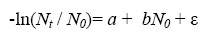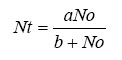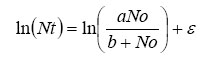### Darren W. Johnson. 2007. Habitat complexity modifies post-settlement mortality and recruitment dynamics of a marine fish. Ecology 88:1716–1725.

Appendix A. Comparison of density-dependent models to describe mortality.

To describe the relationship between recruits and 1-yr old blue rockfish, I compared two functions that are commonly used to describe the stock-recruit relationships of fish. The Ricker and Beverton-Holt functions can both be used to model density-dependent survival from one life stage to the next. However, these models are based on different assumptions and describe slightly different recruitment dynamics. The Ricker model assumes that compensatory mortality depends on the initial density, whereas the Beverton-Holt model assumes that compensatory mortality depends on density of the cohort at each point in time (Fogarty et al. 1991). The Beverton-Holt model describes an asymptotic recruitment function. The Ricker function leads to overcompensating mortality, though overcompensation is not necessarily strong. The Ricker and Beverton-Holt functions are simple models that adequately describe most recruitment functions. Often the available data do not justify the use of more complex models (Hilborn and Walters 2001).

To assess overall model fit for the Ricker function, I fit the following linear transformation of the Ricker model to the data:where Nt = density of 1-yr olds, N0 = density of recruits, a= estimated intercept parameter, b= estimated slope parameter, and ε = residual error . This model assumes a log-normal error structure and was fit to the data using linear regression.

The Beverton-Holt model can be formulated as:where Nt = density of 1-yr olds, N0 = density of recruits, a= maximum number of 1-yr olds surviving, b= the number of recruits needed to produce a/2 1-yr olds, and ε = residual error. To fit the Beverton-Holt model with a lognormal error structure, I fit the following equation to the data using nonlinear regression (Hilborn and Walters 2001):where ε = residual error. The residual error for each the models was used to determine values of Akaike’s information criterion (AIC). Model comparisons based on AIC are as follows.

 Model Residual SS Number of parameters n AIC Beverton-Holt 110.09 2 32 76.4 Ricker 103.7 2 32 74.1

LITERATURE CITED

Fogarty, M. J., M. P. Sissenwine, and E. H. Cohen. 1991. Recruitment variability and the dynamics of exploited marine populations. Trends in Ecology and Evolution 6:241–245.

Hilborn, R., and C. Walters. 2001. Quantitative fisheries stock assessment: Choice, dynamics and uncertainty. Kluwer Academic.

[Back to E088-101]